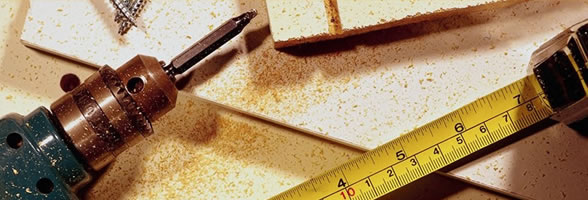DO YOU KNOW THAT ???????

11/08/2013 16:04

The method of getting the square of a number which has the last digit 5 is as follows: Add 1 to the number which is before 5 and multiply it with the same number and add 25.

Example: (75)^2= 7*8=56.

so the required no. is 5625.

—————

Back